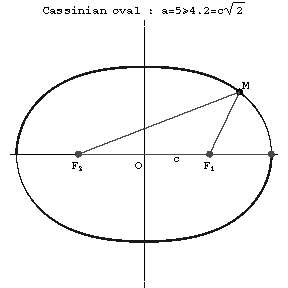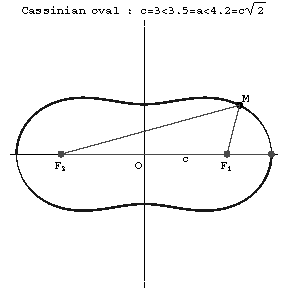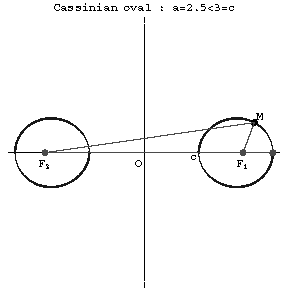Cassini oval

(diff) ← Older revision | Latest revision (diff) | Newer revision → (diff)

A plane algebraic curve of order four whose equation in Cartesian coordinates has the form:

$$(x^2+y^2)^2-2c^2(x^2-y^2)=a^4-c^4.$$Figure: c020700aFigure: c020700bFigure: c020700c

A Cassini oval is the set of points (see Fig.) such that the product of the distances from each point to two given points $F_2=(-c,0)$ and $F_1=(c,0)$ (the foci) is constant. When $a\geq c\sqrt2$ the Cassini oval is a convex curve; when $c<a<c\sqrt2$ it is a curve with "waists" (concave parts); when $a=c$ it is a Bernoulli lemniscate; and when $a<c$ it consists of two components. Cassini ovals are related to lemniscates. Cassini ovals were studied by G. Cassini (17th century) in his attempts to determine the Earth's orbit.